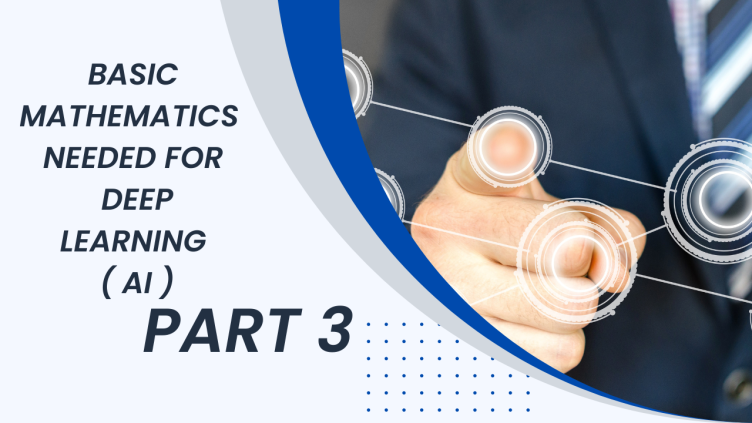# BASIC MATHEMATICS FOR DEEP LEARNING PART 3

Mathematics is the soul of deep learning. Every program developed in deep learning/Machine learning is an implementation of some mathematical model. Basically mathematics is just representation of idea through symbols that's it. In the previous posts we have discusses concept of mathematics such as graph, function, linear equation, Universal approximation theorem which are required in deep learning. If you haven't read that post you can find it here. In this post we will discuss and implement in python . Matrix multiplication and differentiation and also discuss concept of tangent and linear interpolation. Don't afraid because of this big big words because mathematician likes to give big names for simple ideas.

## MATRIX MULTIPLICATION

Matrix multiplication is an operation which is used in matrices. It is used to multiply two matrices. Since matrices are not just one number it is a bunch of numbers that why it follows certain rule to multiply. Lets understand through an example. Let say we have two matrices a and b and we want to multipy it.

a= [1 2]
[4 3]

b= [6 1]
[2 3]

To multipy two matrices. Shape of two matrices follow below rules

1) First matrices column should be equal to second matrices row. Here in above example it is same. As 2 column are there in first matrices and 2 rows are there in second matrices.

Rows = Horizontal number in one line are called Rows

Column = Vertical number in one lines are called column

2) Multiplication Method :- First take first rows of matrices A and multipy and sum B first column which gives first row and first column element of resultant matrices

Likewise every row and every column proceeded to see in action we have really cool website www.matrixmultiplication.xyz. where you find every thing in action. You can cannot reverse multipy matrices to get some answers. Only identity matrices will give you some results. If first matrices column and second matrices rows don't match you cannot multipy two matrices.

## DIFFERENTIATION

As the name suggest it is to calculate difference between two values of dependent variable with respect to independent variable . Let say you want to calculate differentiation of distance with respect to time. Let say you are going from New York to New Jersey and that took you 10 hours to reach there because let say it is 500 Km away and if you want to go somewhere else which 600 km than it would have take you on average 12 hours which shows that time is directly proportional to distance with some constant a

t=a x d where a is constant and d is distance

Where t is time and a is constant and d is distance. If you want to calculate in how much will be lapsed if you covered a very small distance than new time will be tand new distance is dthen there difference will give you tsmall time which is elapsed.

t3 = t-t1

t3 = a(d-d1)

Differentiation actually calculate the tangent of any function .Tangent can be positive or negative it is difference between independent variable by dependent variable. Let say you have function.

y = x2+b

which graph as

Tangents in deep learning are sometimes called gradients which we use to find best model for our problem, and this gradient only decides our model improvements. We use differentiation a lot in deep learning, But beginners don't need to go deep in differentiation because good news is, it is calculated and handle by deep leaning framework. We are only concerns with this tangent. It is also called slope so don't get confuse if you find such terms it only represents change in variable with respect to each other.

## CONCLUSION

In this post we have discussed matrix multiplication and differentiation in some details which required in deep learning. If you want to go deeper than this you can find on mathsisfun com. It is really cool website to learn maths.